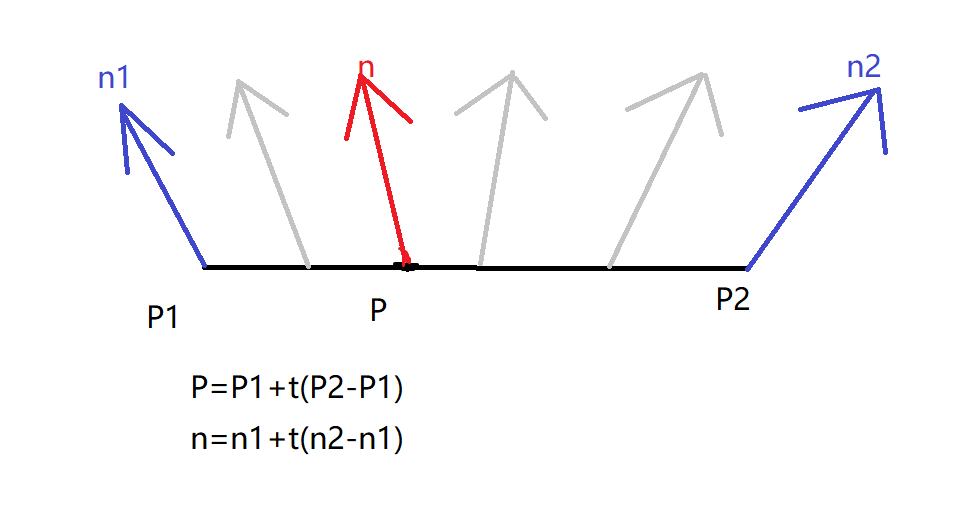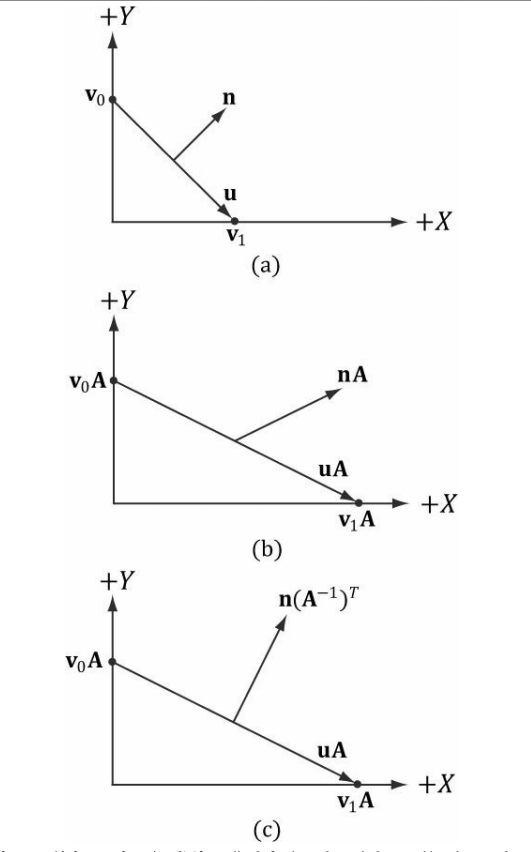2017/10/13

# 顶点法线的概念及其相关计算

## 顶点法线的概念

平面法线是一个平面用来描述其所朝的方向的单位向量，而顶点法线则是一个顶点在某一平面上时的朝向。

这里简单描述一下顶点法线的作用：## 顶点法线的计算

那么我们应如何计算顶点法线呢？我们由定义可知：顶点法线是在平面上的顶点上的，所以我们就可以由要计算的顶点所在的三角形的两条边的叉乘，求出垂直于该三角形所在平面的向量，再将之单位化，即为我们所求的顶点法线，当然，如果这个顶点同时在多个三角形上，要对各个三角形的法向量计算结果求均值

``````	void CalculationNormalVector()
{
for(int i=0;i<m_Vertices.size();i+=3)
{
Vector3D normal=CrossProduct((m_Vertices[i+1].m_Position-m_Vertices[i].m_Position),(m_Vertices[i+2].m_Position-m_Vertices[i+1].m_Position));
m_Vertices[i].m_NormalVector=normal;
m_Vertices[i+1].m_NormalVector=normal;
m_Vertices[i+2].m_NormalVector=normal;
}
bool* flag=new bool[m_Vertices.size()];
memset(flag,false,sizeof(bool)*m_Vertices.size());
for(int i=0;i<m_Vertices.size();i++)
{
if(!flag[i])
{
vector<Vertex*> pointers;
int n=0;
Vector3D sum={0,0,0};
for(int j=i;j<m_Vertices.size();j++)
{
if(m_Vertices[i].m_Position==m_Vertices[j].m_Position)
{
n+=1;
sum=sum+m_Vertices[j].m_NormalVector;
flag[j]=true;
pointers.push_back(&m_Vertices[j]);
}
}
Vector3D ans=sum/n;
for(auto p:pointers)
{
p->m_NormalVector=ans;
}
}
}
delete[] flag;
}
``````

## 法向量的转化

法向量的转化是指将某个平面改变后，如何用改变前的法向量计算出改变后的法向量。上图：设u为平面的上任意两向量的差（也是向量）
设n是平面那个改变前的法向量。
这可知u·v=0 => 两个向量垂直
将其转化为矩阵形式：
u*T(n)=0 T(n)表示n的转置
设A为改变平面的矩阵
所以可得：u* A*I(A)*T(n)=0 I(A)表示A的逆矩阵
因为T(T(A))=AT(A*B)=T(A)*T(B)
所以I(A)*T(n)=n*T(I(A))
所以可列出：
u*A·n*T(I(A))=0
设B=T(I(A))

n*B即为变换后的平面的法向量了。

在实际的应用中我们往往会在Vertex Shader中使用这一矩阵(B)来计算经过世界变换后的物体的顶点上的顶点法线，这时的矩阵A即是我们物体的世界变换矩阵。

Show Disqus Comments

### welcome to visit my github